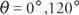## Trigonometric Equations

Trigonometric identities are true for all replacement values for the variables for which both sides of the equation are defined. Conditional trigonometric equations are true for only some replacement values. Solutions in a specific interval, such as 0 ≤ x ≤ 2π, are usually called primary solutions. A general solution is a formula that names all possible solutions.

The process of solving general trigonometric equations is not a clear‐cut one. No rules exist that will always lead to a solution. The procedure usually involves the use of identities, algebraic manipulation, and trial and error. The following guidelines can help lead to a solution.

If the equation contains more than one trigonometric function, use identities and algebraic manipulation (such as factoring) to rewrite the equation in terms of only one trigonometric function. Look for expressions that are in quadratic form and solve by factoring. Not all equations have solutions, but those that do usually can be solved using appropriate identities and algebraic manipulation. Look for patterns. There is no substitute for experience.

Example 1: Find the exact solution: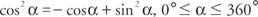First, transform the equation by using the identity sin 2 α + cos2α = 1.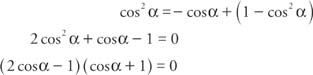Therefore,Thus,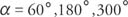Example 2: Solve cos 2 x = 3(sin x − 1) for all real values of x.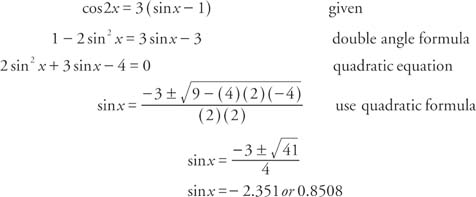The first answer, −2.351, is not a solution, since the sine function must range between − 1 and 1. The second answer, 0.8508, is a valid value. Thus, if k is an integer,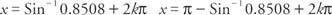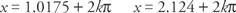In degree form,Example 3: Find the exact solution: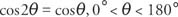First, transform the equation by using the double angle identity cos 2θ = 2 cos 2θ − 1.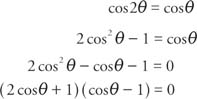Therefore,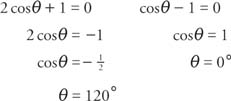Thus,